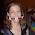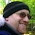## Monday, October 09, 2006

### Seven Ate Nine

Can you replace the missing digits in the number 789,XYZ so that the resulting number is divisibile by 7, 8 and 9? The only restriction being you can't use a 7,8, or 9.

1.Not so tough, I don't think.

X+Y+Z = 3 or 12 or 21, but 21 is too high (requires a 7, 8 or 9).

If X + Y + Z = 3, then we have something with three 1's, but that is no good for divisibility by 8, or a 0, a 1, and a 2, but numbers ending in 012 are not divisible by 8 either and 789,120 is not divisible by 7. Therefore X+Y+Z = 12.

Choose from the following list:
660, 651, 642, 633, 552, 543, 444.

The arrangements that are divisible by 8 are: 264, 336, 552 and 624.

789 - 264 = 525, bingo
789 - 336 = 453, not divisible by 7.
789 - 552 = 237, not divisible by 7.
789 - 624 = 165, not divisible by 7.

So, 789,264 =
9*87,696 =
8*98,658 =
7*112,752

2.dang, you barely beat me to a similar explanation. one addition to your explanation (which is pretty obvious maybe): the reason you need X+Y+Z=12 is so the total 7+8+9+X+Y+Z will be divisible by 9 and so will the whole number (since every number divisible by 9 has the sum of its digits divisible by 9 also). also, you know 1000 is divisible by 8, and so is 789,000, which is why you can just look at the last three digits for divisibility by 8.

3.The explanations are skipping a lot of steps, I think. Mike--will you provide a full one?

4.I'm afraid I have a very simple answer to this one. Note that 7x8x9 = 504. Divide 789,000 by 504 you get a remainder of 240. 540-240 = 264. So 789,264 is one of the possible numbers. The only other number divisible by 504 is 789,768, which repeats the 7 and 8 which were restricted.

Leave your answer or, if you want to post a question of your own, send me an e-mail. Look in the about section to find my e-mail address. If it's new, I'll post it soon.

Please don't leave spam or 'Awesome blog, come visit mine' messages. I'll delete them soon after.

Enter your Email and join hundreds of others who get their Question of the Day sent right to their mailbox

The Lamplight Manor Puzz 3-D